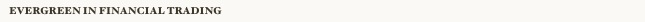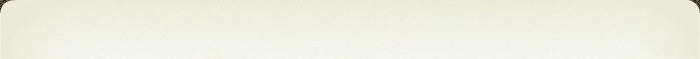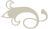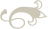Evergreen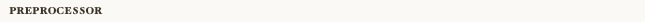The Scheme implementation is a MAXSAT-solver booster that n-maps CNF formulae with respect to look-ahead polynomials, and the Java implementation is a MAXCSP-solver booster that n-maps CSP formulae with respect to look-ahead polynomials.

Scheme Implementation, Version 0.7 (source)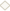Binary for MAC OS X (Intel), README
Java Implementation, Version 0.7 (source, README)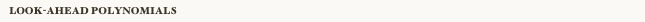Given a set of pairs of relations and weights, the lookahead polynomials predicts the fraction of satisfied constraints in your formula. The prediction is 100% accurate when the formula is fully symmetric.
Version 0.2 (source)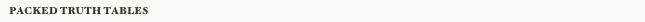An efficient representation of truth tables of Boolean relations such as: OR(x,y,z,w,t), 1in3(x,y,z). The whole truth table is represented as a single 32 bit integer. Therefore, it can represent up to rank 5 relations. The representation provides the following operations: restriction (we call it reduction), forced variable identification, irrelevant variable detection, n-mapping (negating a variable), renaming (permutation), flipping variables, generating Qs's (which is needed for the abstract representation). All of these operations have extremely efficient implementations using bitwise manipulations.
Version 2.0 (source, doc)
Version 1.1 (source)